Video Tutorial Born-Haber Cycles

Quick Notes Born-Haber Cycles

• Born-Haber cycles are used to find lattice enthalpies indirectly using experimental data.
• It is impossible to directly measure lattice enthalpies experimentally, this is why Born-Haber cycles have to be used.
• Hess’ Law states that the overall enthalpy change for a given reaction is the same, regardless of the route taken to form the product (at the same temperature and pressure).
• Born-Haber cycles are made by using two different routes to form an ionic lattice from its constituent elements in their standard states.
•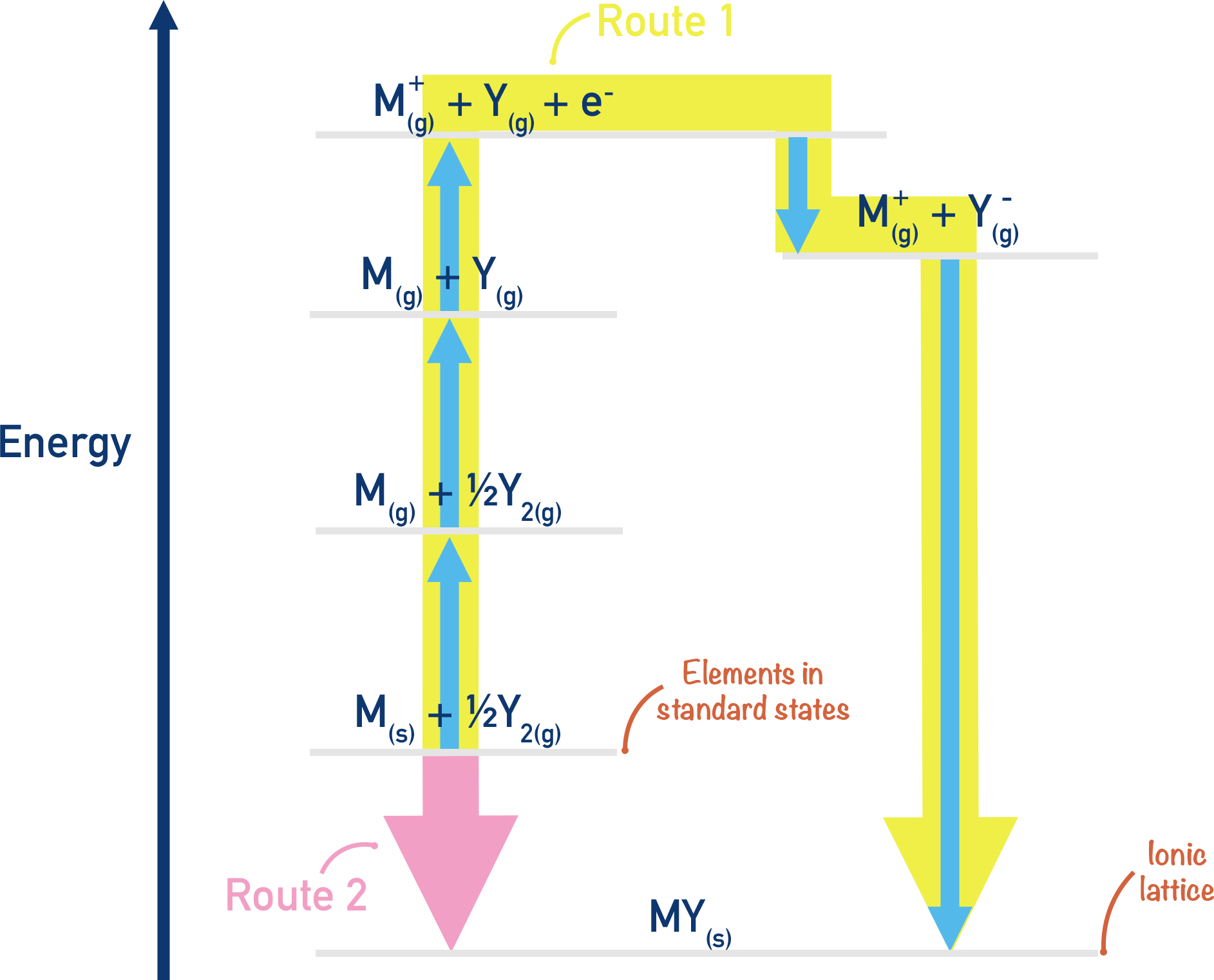• Different standard reactions are used to construct a Born-Haber cycle.
• Standard enthalpy of atomisation – enthalpy change when one mole of gaseous atoms is formed from its element (in its standard state).
• (First) Ionisation energy – enthalpy change when one moles worth of electrons are removed from one mole’s worth of gaseous atoms.
• (First) Electron affinity – enthalpy change when one electron is added to each atom in one mole’s worth of gaseous atoms.
• Standard enthalpy of formation – enthalpy change when one mole of a compound is formed from its constituent elements under standard conditions.

Full Notes Born-Haber Cycles

It is impossible to directly measure lattice enthalpies experimentally. Separate samples of gaseous positive and negative ions would need to be kept apart, then carefully mixed and the released thermal energy measured (see Lattice Enthalpies).

Instead, we find lattice enthalpies indirectly by applying Hess’ Law (see Hess Cycles). An energy cycle is constructed using two different routes to form a particular lattice from its constituent elements. Constituent elements refer to the elements that ‘make-up’ the lattice (for example, the constituent elements of magnesium chloride are magnesium and chlorine). The energy cycle is then used to find the lattice enthalpy and is referred to as a Born-Haber cycle.

## Standard Enthalpies

There are some ‘standard’ reactions involved in a Born-Haber cycle, exam boards require you to know these! Also, remember that standard enthalpy changes always refer to enthalpy change per mole.

Reaction Definition Exothermic or Endothermic
Standard enthalpy change of formation, ΔHf Enthalpy change when one mole of a compound (in its standard state) is formed from its constituent elements (in standard states) Exothermic (negative enthalpy change, -ΔH)
Standard enthalpy change of atomisation, ΔHat Enthalpy change when one mole of gaseous atoms is formed from its element (in its standard state) Endothermic (positive enthalpy change, +ΔH)
First ionisation energy, ΔHI.E Enthalpy change when one moles worth of electrons are removed from one mole’s worth of gaseous atoms Endothermic (positive enthalpy change, +ΔH)
First electron affinity, ΔHE.A Enthalpy change when one electron is added to each atom in one mole’s worth of gaseous atoms Exothermic (negative enthalpy change, -ΔH)

## Born-Haber Cycle for Sodium Chloride, NaCl

For the following example, we will be referring to the formation of sodium chloride (NaCl).

For more complicated lattices, please see the notes at the bottom of the page on second and third ionisation energies and second electron affinities

There are two ways we can form the ionic lattice of sodium chloride, NaCl(s), from its constituent elements.

Route 1:

The easiest way is to take the elements that make up sodium chloride (NaCl) in their standard states (Sodium - Na(s) and chlorine - Cl2(g)) and simply react them together. This is a straightforward reaction and the enthalpy change is easy to measure.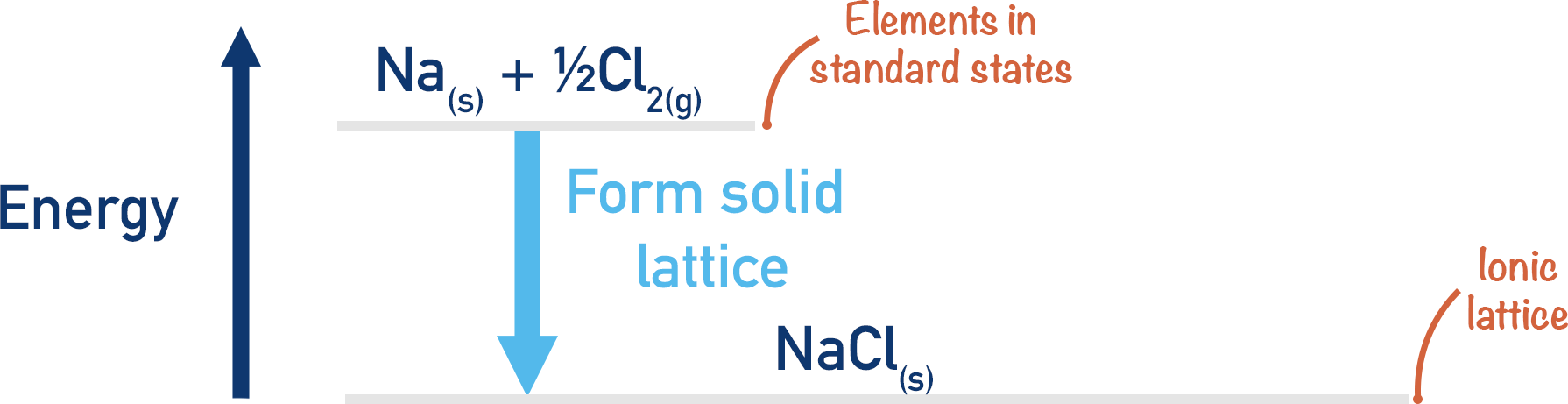This process would be exothermic (energy would be released), meaning it would have a negative enthalpy change (-ΔH).

Na(s) + ½Cl2(g) → NaCl(s)

Note, although we are forming a lattice here, the enthalpy change is not a lattice enthalpy as the lattice is not being formed from gaseous ions, just the constituent elements in their standard states (). This makes the enthalpy change a ‘standard enthalpy of formation’.

Route 2:

Another way to form the lattice would be to convert the sodium and chlorine into gaseous ions, then react these gaseous ions together to form the lattice, NaCl(s). This method would involve several steps as it is not possible to simply go from Na(s) + ½Cl2(g) → Na+(g) + Cl-(g) in one go! These steps can be linked together to form an energy cycle, as outlined in detail below.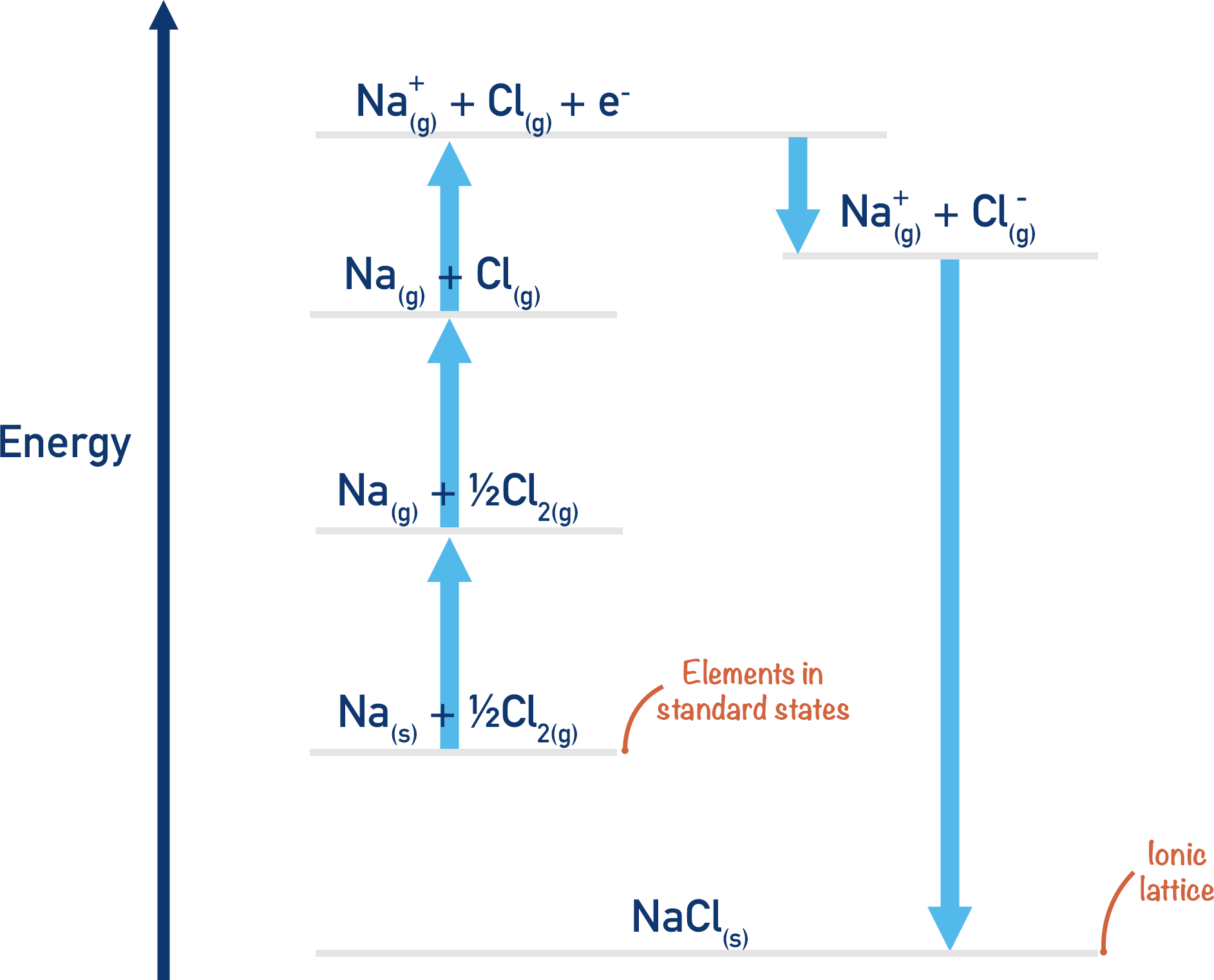The Born-Haber cycle is a way of drawing out the two possible ways we can go from Na(s) + ½Cl2(g) to NaCl(s).

## Atomisation

Firstly, both the sodium and the chlorine have to be ‘atomised’ and turned into single, free gaseous atoms. This means breaking apart their bonds to other atoms. For sodium, this would be overcoming the bonding in the metallic structure of solid sodium metal. For chlorine, this would be breaking the covalent bonds between chlorine atoms in chlorine molecules (Cl2).

We can measure the enthalpy change for the atomisation of both sodium and chlorine, but not at the same time. We have to break this stage down into two steps.

Na(s) + ½Cl2(g) → Na(g) + ½Cl2(g)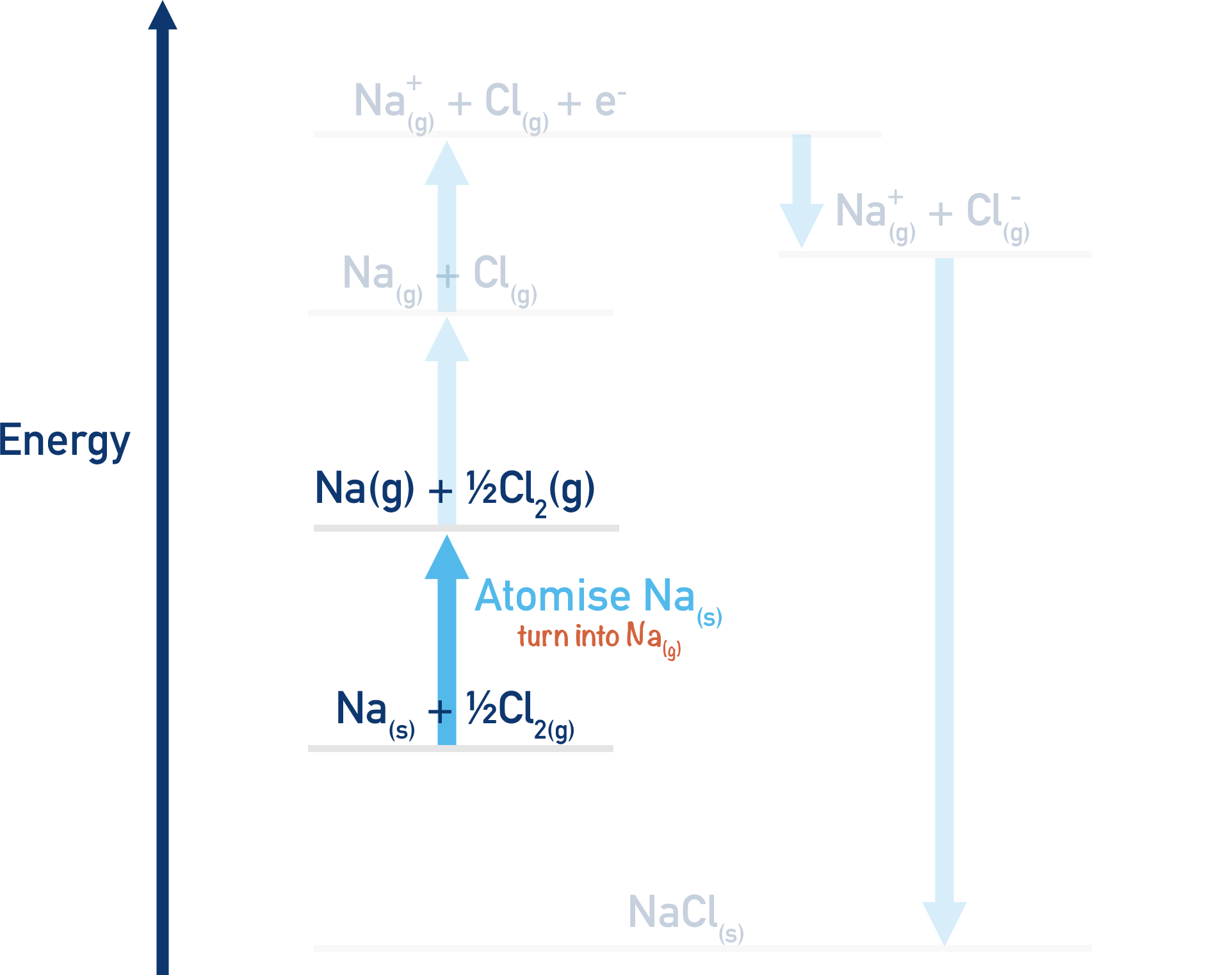Here, we have atomised the sodium as it has gone from Na(s) to Na(g). Energy is needed for this process (endothermic), it will have a positive enthalpy change (+ΔH).

Na(g) + ½Cl2(g) → Na(g) + Cl(g)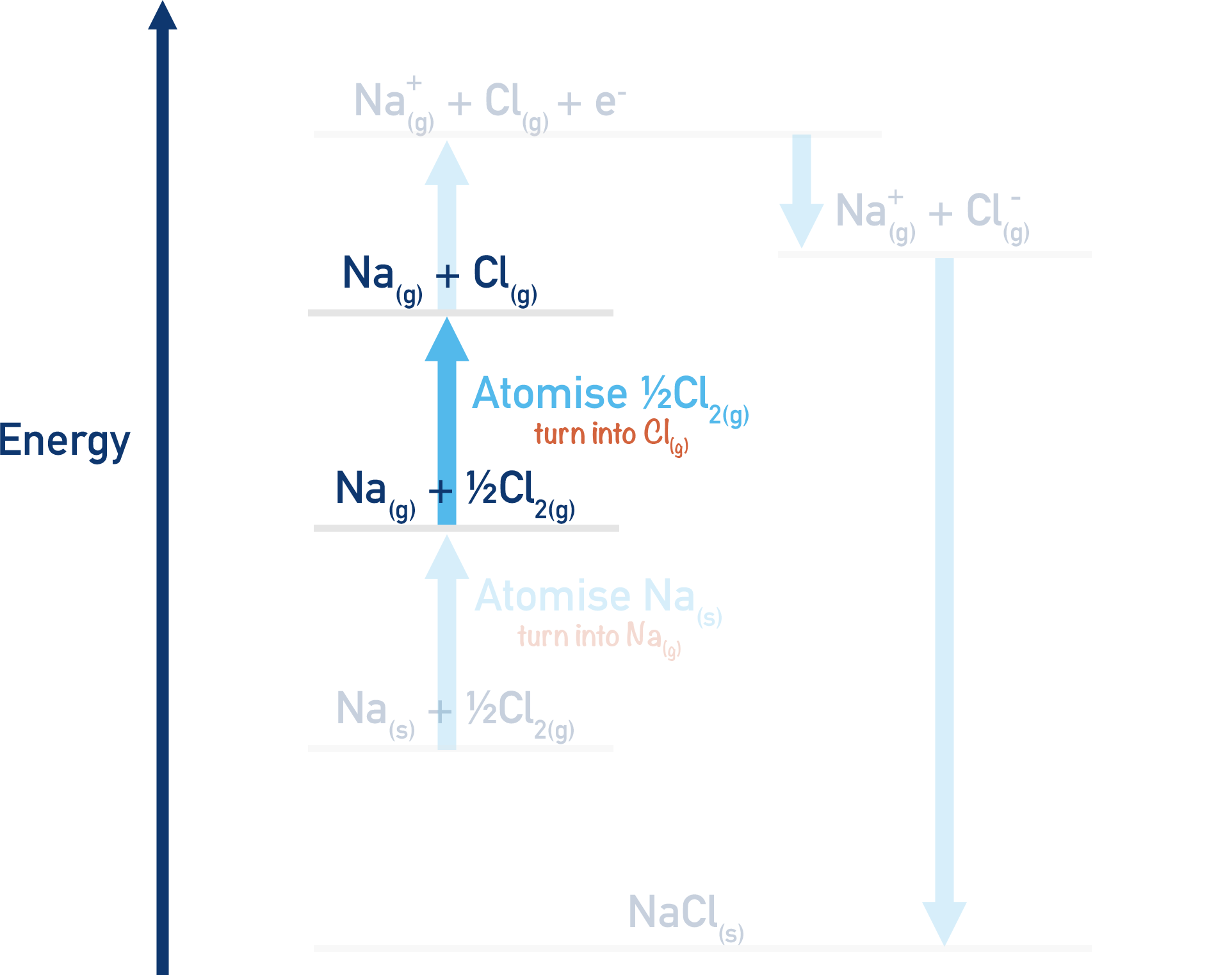Here, we have atomised the chlorine as it has gone from ½Cl2(g) to Cl(g). Energy is needed for this process (endothermic), it will have a positive enthalpy change (+ΔH).

## Ionisation

Now we have converted the Na(s) and ½Cl2(g) into gaseous atoms, the only remaining thing to do is convert them into charged ions. We have to measure the enthalpy changes for converting both atoms to ions independently.

Na(g) + Cl(g) → Na+(g) + Cl(g) + e-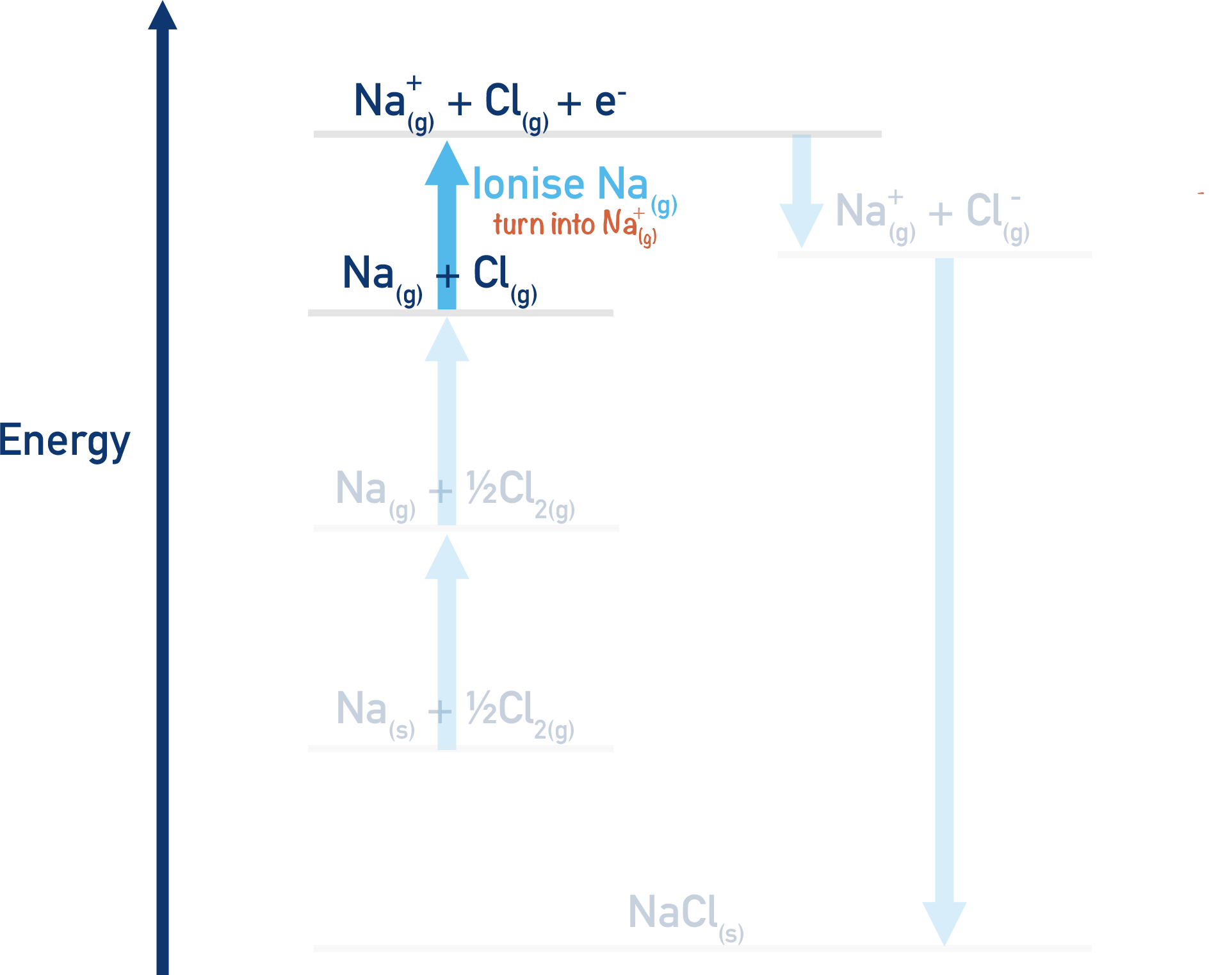Here, the gaseous sodium atom has been converted into a positive ion by the removal of one electron. This is the same as the first ionisation energy of sodium (). The e- on the right-hand side of the equation comes from the sodium atom. Energy is needed for this process (endothermic), it will have a positive enthalpy change (+ΔH).

Na+(g) + Cl(g) + e- → Na+(g) + Cl-(g)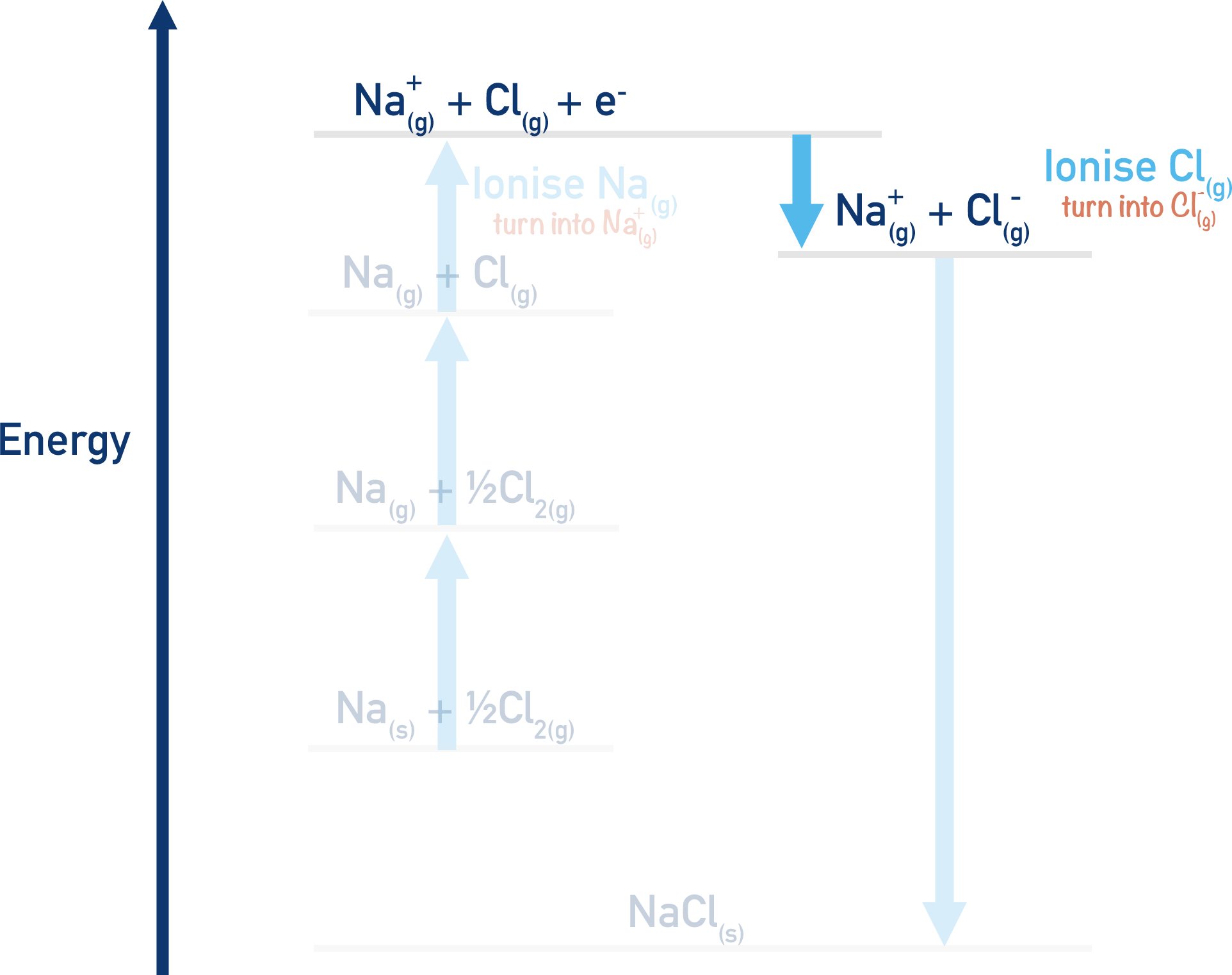Here, the gaseous chlorine atom has been converted into a negative ion by the addition of one electron. This is referred to as ‘first electron affinity’. This process would actually release energy (exothermic) and have a negative enthalpy change (-ΔH). This is because the chlorine atom would be far more stable as a negatively charged ion.

## Lattice Enthalpies of Formation

Now we have the species Na+(g) and Cl-(g). The final step in this process would be mixing these two together to form the ionic lattice NaCl(s).The enthalpy change for this part of the process is impossible to measure experimentally, although we know the enthalpy change would be negative (). This is where the Hess Cycle comes in.

## Overall Born-Haber Cycle

We have outlined two possible routes above to go from Na(s) + ½Cl2(g) to NaCl(s) and have a complete cycle.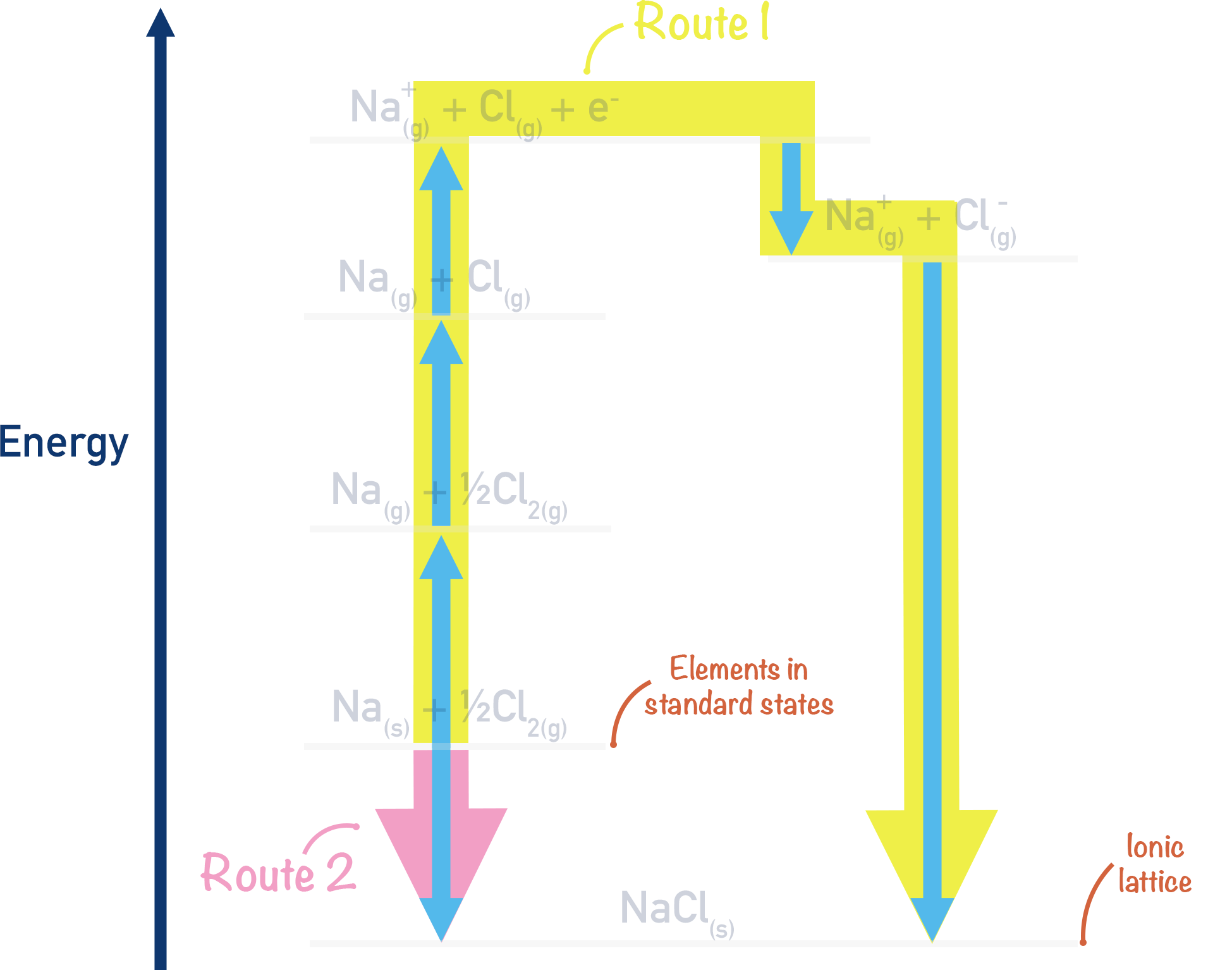By putting ‘actual’ values in for each stage in the two routes, we can find the lattice enthalpy of NaCl using Hess Law.

The values used are ‘standard enthalpy’ changes (see above) and should be provided in an exam. They are ‘data book’ values, which have been found by carrying out each type of reaction under standard conditions (with molar quantities). By using these values, it is unnecessary to actually carry out the reactions in the Born-Haber cycle each time we want to find a lattice enthalpy!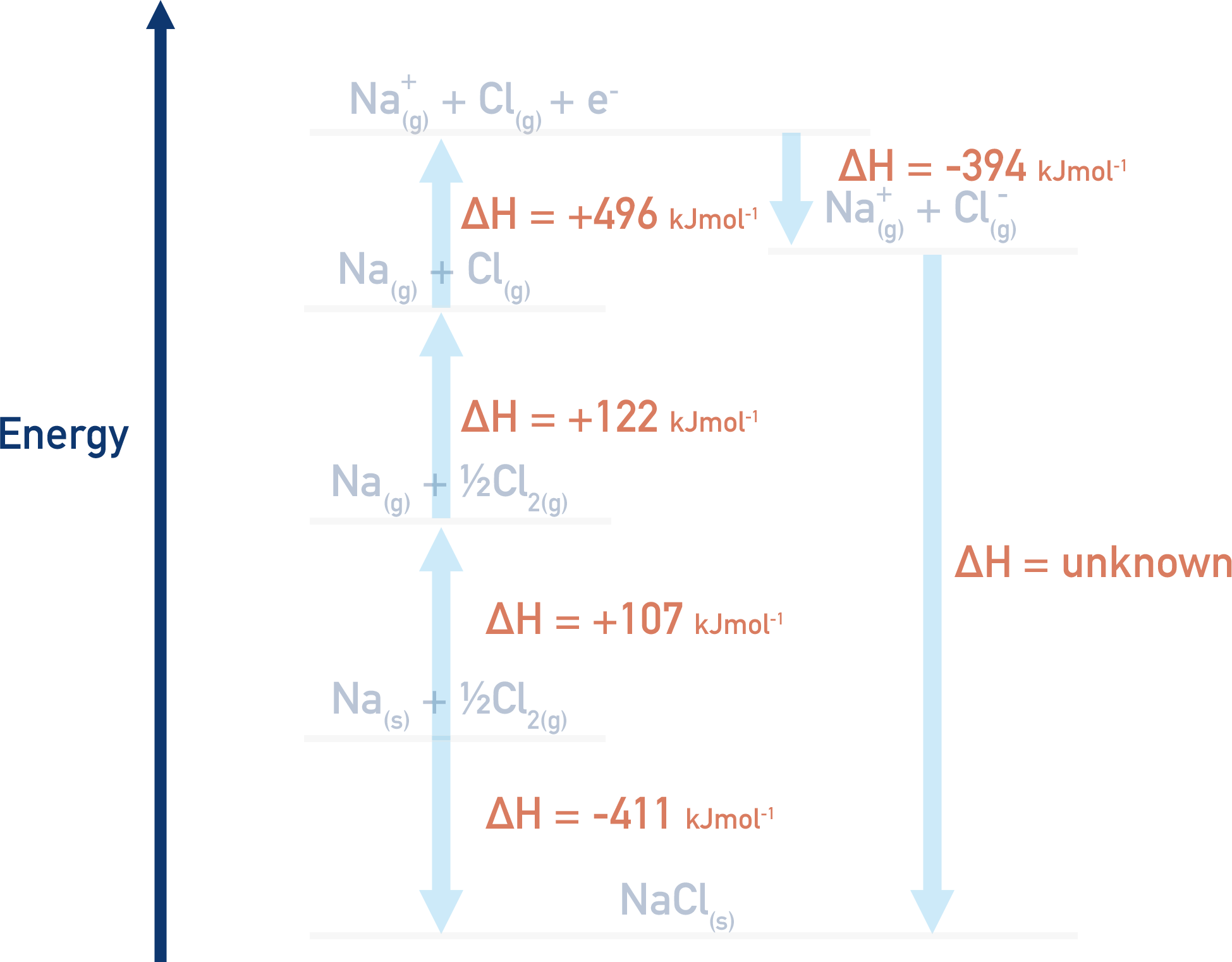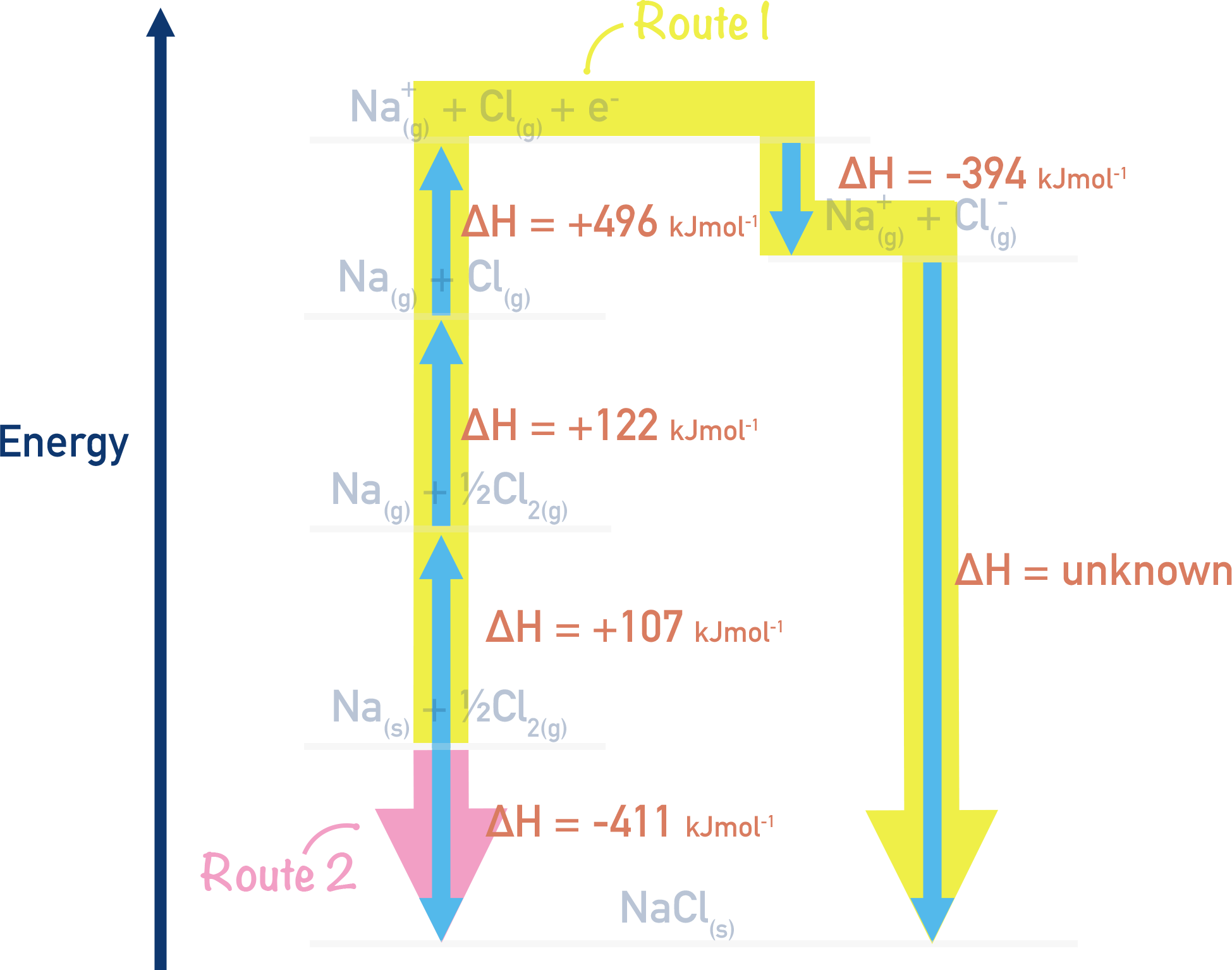After the values are put into the cycle, we can follow Hess’ Law to find the value of lattice enthalpy. Remember route 1 = route 2 (see Hess Cycles).

## Using Hess’ Law:

Route 1 = Route 2

Route 1 = (+107) + (+122) + (+496) + (-394) + ΔH

Route 2 = (-411)

This means

(+107) + (+122) + (+496) + (-394) + ΔH = -411

+ 378 + ΔH = -411

Rearrange to give

ΔH = (-411) – (+378)

ΔH = -789 kJmol-1

Note:

All the + and – signs make things look complicated, but I strongly recommend you put the signs in brackets to make sure your enthalpy changes are correct! Otherwise, things can get very messy. You may be taught to ‘reverse the sign’ of an enthalpy change to go in a different direction in the cycle. This method will work, but, in my experience, it means there is a far greater chance of getting your final value wrong! By copying the values as given in a data table, you can simply follow your cycle. Put them in brackets though! This helps avoid addition errors with your calculator.

## For ions with a charge greater than 1+ or 1-

Many ionic lattices are made up of ions with a charge that is greater than 1+ or 1-. To form such ions, successive ionisation energies and successive electron affinities are needed.

This is why some Born-Haber cycles may have more steps in than others.

For example...

Aluminium Chloride, AlCl3 is made up of Al3+ ions.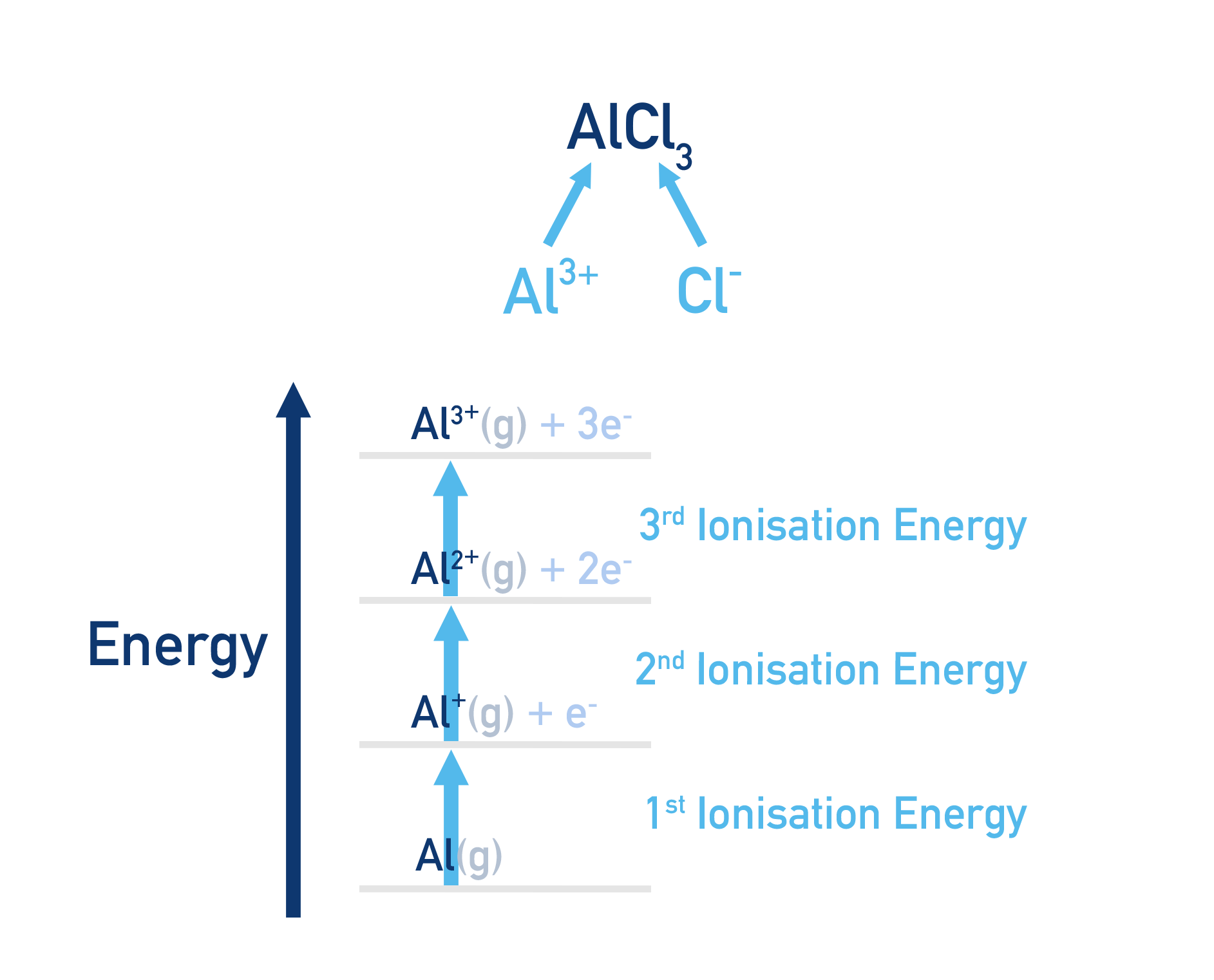To form 3+ ions, the aluminium has to be ionised three times. First ionisation energy, second ionisation energy and third ionisation energy. These are called successive ionisation energies.

These steps have to be carried out independtly and then added together to find the overal energy needed to go from Al to Al3+.

Oxygen forms O2- charged ions.

To form a charge of 2-, an oxygen atom must have one electron added (first electron affinity) and then a second electron added to the O- ion (second electron affinity) to form an O2- ion.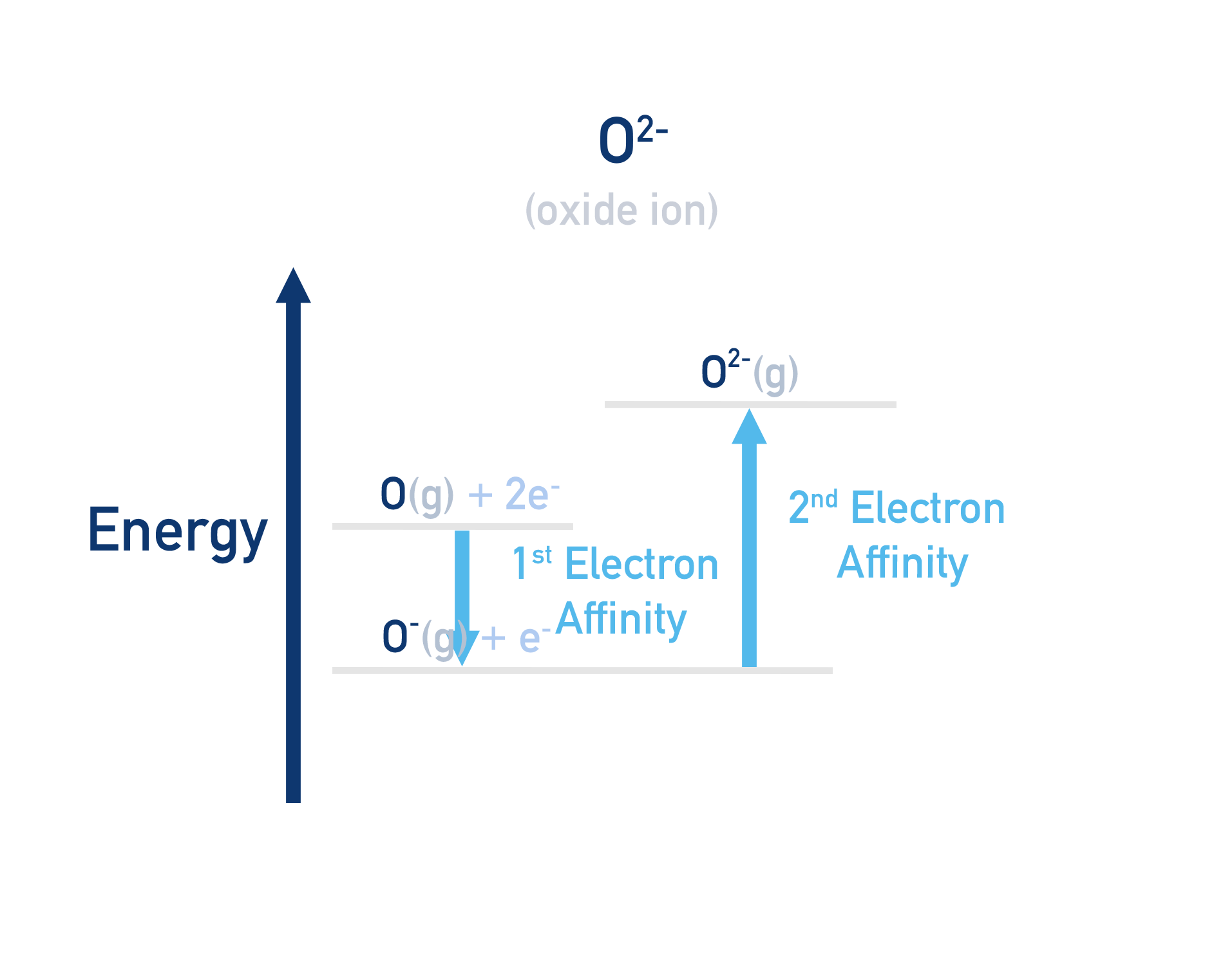Note that the second electron affinity for oxygen (and any element) is positive. This is because it takes energy to overcome the repulsion between a negatively charged ion (O-) and the incoming electron (e-).